# MA.912.AR.2.6Export Print
Given a mathematical or real-world context, write and solve one-variable linear inequalities, including compound inequalities. Represent solutions algebraically or graphically.

### Examples

Algebra 1 Example: The compound inequality 2x≤5x+1<4 is equivalent to -1≤3x and 5x<3, which is equivalent to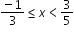General Information
Subject Area: Mathematics (B.E.S.T.)
Strand: Algebraic Reasoning
Status: State Board Approved

## Benchmark Instructional Guide

### Terms from the K-12 Glossary

• Linear Expression

### Vertical Alignment

Previous Benchmarks

Next Benchmarks

### Purpose and Instructional Strategies

In grade 8, students solved two-step linear inequalities in one variable. In Algebra I, students solve one-variable linear inequalities, including compound inequalities. In later courses, students will solve problems involving linear programming and will solve one-variable quadratic inequalities.
• Instruction emphasizes that solutions for compound inequalities with an or statement contain all the solutions for both inequalities and that solutions for compound inequalities with an and statement contain only the solutions that both inequalities have in common. Allow for student discovery of this by discussing whether each point on a number line is a solution for its compound inequality (MTR.4.1).
• For example, plot various points on a number line and ask student whether certain points are a solution to the compound inequality $x$ < −6 or $x$ ≥ 8.
• Instruction includes student understanding that compound inequalities with or create a combining (or a $u$$n$$i$$o$$n$) of the solutions of the individual inequalities while compound inequalities with $a$$n$$d$ create an overlap (or an $i$$n$$t$$e$$r$$s$$e$$c$$t$$i$$o$$n$) of the solutions of the individual inequalities.
• For mastery of this benchmark, students do not need to have familiarity with the terms “unions” and “intersections” as this will be part of a later course.
• Instruction builds understanding that compound inequalities may have different looks graphically.
• Graphs of Compound Inequalities with $o$$r$
• $x$ ≥ 6 $o$$r$ $x$ ≤ 1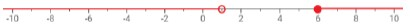• $x$ < −5 $o$$r$ $x$ ≥ −9 (Solution is all real numbers)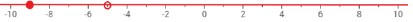• $x$ ≥ 3 $o$$r$ $x$ > 0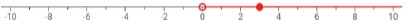• Graphs of Compound Inequalities with $a$$n$$d$
• $x$ ≥ 6 $a$$n$$d$ $x$ ≤ 1 (There are no solutions)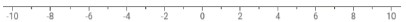• $x$ < −5 $a$$n$$d$ $x$ ≥ −9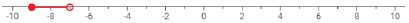• $x$ ≥ 3 $a$$n$$d$ $x$ > 0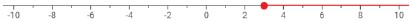### Common Misconceptions or Errors

• Given that students’ prior experience has dealt with equation work far more than inequality work, students will likely forget to consider the direction of the inequality symbol as they solve inequalities. To address this, have these students consider a context to understand the need to pay attention to the direction of the inequality symbol.
• When creating inequalities, look for students to experience confusion with the phrase “less than.” Students interpreting the phrase “eight less than four times a number” (4$n$ − 8) could incorrectly model it as 8 – 4$n$ or 8 < 4$n$
• For students writing 8 – 4$n$, restate the phrase and then ask “how much less than 4$n$?” to prompt them to realize that 8 should be subtracted from $n$
• For students writing 8 < 4$n$, highlight the importance of the word “is” in signaling an inequality; “eight is less than four times a number.” The word “is” has played an important role in equation contexts as well, so it should be an easy transition for students to consider it for inequalities.

### Strategies to Support Tiered Instruction

• Teacher models which direction to shade when graphing inequalities. Teacher additionally models and co-creates a number line while providing a think-aloud explaining that after solving for $x$, you must select values for $x$. If the value selected creates a true statement, have students place a dot on that value. If it creates a false statement, have students place an “$x$” on that value. At the beginning, students may need to choose a few values before being able to accurately shade. The same thing can be done for compound inequalities by replacing values for $x$ in both inequalities.
• Teacher provides instruction involving real-world contexts where students must determine the direction of the inequality symbol on a number line.
• Teacher supports student understanding on why the inequality symbol changes direction when multiplying or dividing by a negative.
• For example, students are provided with a numerical inequality and a series of operations where they put in the inequality after each operation to determine when the inequality is flipped and when it is not.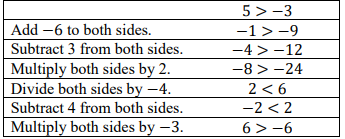• Teacher provides a highlighter to identify the phrases “is less than,” “is greater than,” “is less than or equal to,” and “is greater than or equal to” when writing inequalities.
• Instruction includes the use of algebra tiles to model the distributive property or to add and subtract like terms as a problem is solved algebraically.
• An example using algebra tiles for the expression −2(2$x$ − 3) is shown below.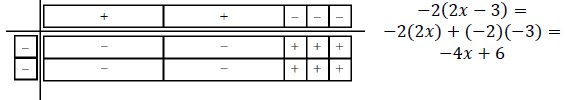• An example using algebra tiles for the expression −3$x$ + 2 + 5$x$ − 6 + 5 is shown below.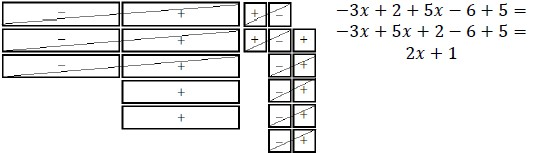• Instruction includes providing expressions and have students act out the context with props.
• For example, Karen earns a base salary of \$3500 plus \$200 commission for each sale made. She wants to earn at least \$4500 this month, and the company will only pay at most \$5300 each month. In this example, give a student play money and then ask how much money they make after 1 sale, 2 sales, and so on this month. Then ask them what the least number of sales they want to make and the most number of sales they would want to make, and to represent this using an inequality.
• Instruction includes vocabulary development by co-creating a graphic organizer for each property of inequality.

• Each month, Phone Company A charges \$105 for unlimited talk and text plus an additional \$11.50 for each gigabit of data used. Phone Company B charges \$28.50 per gigabit of data used, and the price includes unlimited talk and text.
• Part A. How many gigabits of data could a person use monthly to make the total cost from Phone Company B more expensive than Phone Company A?
• Part B. Compare your solution with a partner.
• Part C. What are all of the possible gigabits a person could use to make the total cost from Phone Company B more expensive than Phone Company A?

• Tamar is shopping for food for an upcoming graduation party for her senior class. She starts with \$350 and hopes to retain between \$100 and \$125 after her purchases to hire a DJ. She spends a total of \$163 on food and plans to buy 24-packs of soda that are priced at \$8 each. What range of 24-packs of soda can Tamar afford to buy?

### Instructional Items

Instructional Item 1
• The expression 45$h$ + 225 represents the total cost in dollars of renting a large party tent from a local rental store for h hours. Jamal’s parents are willing to spend between \$500 and \$750 for the tent.
• Part A. Write an inequality to represent the possible lengths of time the tent can be rented.
• Part B. Using your inequality in Part A, what is one possible length of time the tent could be rented? Give your answer in number of hours.

Instructional Item 2
• Graph the solution to the inequality −$\frac{\text{4}}{\text{5}}$ − 9.2$w$ < 1.24$w$ − 3.75.

*The strategies, tasks and items included in the B1G-M are examples and should not be considered comprehensive.

## Related Courses

This benchmark is part of these courses.
1200310: Algebra 1 (Specifically in versions: 2014 - 2015, 2015 - 2022, 2022 and beyond (current))
1200320: Algebra 1 Honors (Specifically in versions: 2014 - 2015, 2015 - 2022, 2022 and beyond (current))
1200370: Algebra 1-A (Specifically in versions: 2014 - 2015, 2015 - 2022, 2022 and beyond (current))
1200400: Foundational Skills in Mathematics 9-12 (Specifically in versions: 2014 - 2015, 2015 - 2022, 2022 and beyond (current))
7912080: Access Algebra 1A (Specifically in versions: 2014 - 2015, 2015 - 2018, 2018 - 2019, 2019 - 2022, 2022 and beyond (current))
1200315: Algebra 1 for Credit Recovery (Specifically in versions: 2014 - 2015, 2015 - 2022, 2022 and beyond (current))
1200375: Algebra 1-A for Credit Recovery (Specifically in versions: 2014 - 2015, 2015 - 2022, 2022 and beyond (current))
7912075: Access Algebra 1 (Specifically in versions: 2014 - 2015, 2015 - 2018, 2018 - 2019, 2019 - 2022, 2022 and beyond (current))
1209315: Mathematics for ACT and SAT (Specifically in versions: 2022 and beyond (current))

## Related Access Points

Alternate version of this benchmark for students with significant cognitive disabilities.
MA.912.AR.2.AP.6: Given a mathematical and/or real-world context, select a one-variable linear inequality that represents the solution algebraically or graphically.

## Related Resources

Vetted resources educators can use to teach the concepts and skills in this benchmark.

## Formative Assessments

Solving a Multistep Inequality:

Students are asked to solve a multistep inequality.

Type: Formative Assessment

Solve for Y:

Students are asked to solve a linear inequality in one variable.

Type: Formative Assessment

Music Club:

Students are given a real world context and asked to model the situation by writing and then solving a multistep inequality.

Type: Formative Assessment

## Original Student Tutorials

Solving Inequalities and Graphing Solutions Part 2:

Learn how to solve and graph compound inequalities and determine if solutions are viable in part 2 of this interactive tutorial series.

Type: Original Student Tutorial

Solving Inequalities and Graphing Solutions: Part 1:

Learn how to solve and graph one variable inequalities, including compound inequalities, in part 1 of this interactive tutorial series.

Type: Original Student Tutorial

Writing Inequalities with Money, Money, Money:

Write linear inequalities for different money situations in this interactive tutorial.

Type: Original Student Tutorial

## MFAS Formative Assessments

Music Club:

Students are given a real world context and asked to model the situation by writing and then solving a multistep inequality.

Solve for Y:

Students are asked to solve a linear inequality in one variable.

Solving a Multistep Inequality:

Students are asked to solve a multistep inequality.

## Original Student Tutorials Mathematics - Grades 9-12

Solving Inequalities and Graphing Solutions Part 2:

Learn how to solve and graph compound inequalities and determine if solutions are viable in part 2 of this interactive tutorial series.

Solving Inequalities and Graphing Solutions: Part 1:

Learn how to solve and graph one variable inequalities, including compound inequalities, in part 1 of this interactive tutorial series.

Writing Inequalities with Money, Money, Money:

Write linear inequalities for different money situations in this interactive tutorial.

## Student Resources

Vetted resources students can use to learn the concepts and skills in this benchmark.

## Original Student Tutorials

Solving Inequalities and Graphing Solutions Part 2:

Learn how to solve and graph compound inequalities and determine if solutions are viable in part 2 of this interactive tutorial series.

Type: Original Student Tutorial

Solving Inequalities and Graphing Solutions: Part 1:

Learn how to solve and graph one variable inequalities, including compound inequalities, in part 1 of this interactive tutorial series.